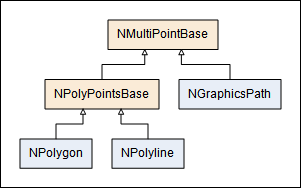﻿ Multipoint Geometries
Nevron Open Vision Documentation
Framework / Graphics / Geometries / Multipoint Geometries
In This Topic
Multipoint Geometries
In This Topic

In the the Primitive Geometries we discussed the primitive geometry objects, that are usually defined by a finite set of points or by simple mathematical equasions. In graphics however you often have to deal with geometry objects that are constructed by multiple points, such as polylines, polygons and paths. In NOV graphics the base class for such objects is the NMultiPointBase class, that serves as base class for the NGraphicsPath, NPolygon and NPolyline as shown by the dollowing class diagram:The rest of the topic talks about the NMultiPointBase features that are common for graphics paths and polygons and polylines.

NMultiPointBase

The common features of the NGraphicsPath, NPolygon and NPolyline objects are implemented by the NMultiPointBase. These include:

• Bounding - you can get the bounding of the object via the Bounds or the BoundsRange properties.
• Query for Points Count - you can get the points count by the GetPointCount() method;
• Get and Set specific points - you can get and set specific point at a certain index via the GetPoint and SetPoint methods.

The NMultiPointBase class derives from the NSealableObject class, meaning that you can prevent further modifications on any NGraphicsPath, NPolygon and NPolyline by calling its Seal() method.
You can query whether a sealable object is sealed via its IsSealed property.

NPolygon and NPolyline

A polygon is flat closed figure that is bounded by a finite set of straight line segments, that form a loop. The following code creates a sample polygon.

Creating a Polygon
Copy Code
```NPolygon polygon = new NPolygon();
```

A polygon can be either convex or concave. A convex polygon is defined as a polygon with all its interior angles less than 180°. This means that all the vertices of the polygon will point outwards, away from the interior of the shape. Think of it as a 'bulging' polygon. Note that a triangle (3-gon) is always convex. The NPolygon-IsConvex property can help you determine whether a polygon is convex.

A polyline is a flat open figure that represents a connected series of straight line segments. The following code creates a sample polyline:

Creating a Polyline
Copy Code
```NPolyline polyline = new NPolyline();